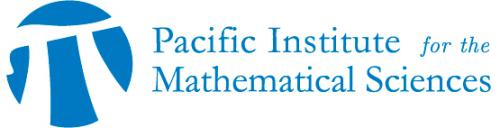## PIMS-ULethbridge Distinguished Speaker Series

• Date: 11/10/2017
Speaker(s):
Jason Bell, University of Waterloo
Location:

University of Lethbridge

Topic:

Linear recurrences, dynamics, and finite-state machines

Description:

Given a field K, a K-valued sequence is said to satisfy a linear recurrence if the n-th term can be expressed as a fixed K-linear combination of the preceding d terms for some d. The sequence of Fibonacci numbers is a famous example of such a sequence. Given a linearly recurrent sequence f(n), the problem of determining the set of natural numbers n where f(n)=0 has a long and very interesting history. In characteristic zero there is a beautiful characterization of which sets can occur due to Skolem, Mahler, and Lech. We show how this problem can be cast in the more general framework of dynamical systems and look at the corresponding dynamical problems; we also explore connections to the theory of finite-state machines, which we show arise naturally when one instead works in positive characteristic.

2017_Nov_10_-_DS_poster.pdf
Schedule:

Time: 12:00-12:15pm

Location: C630 University Hall